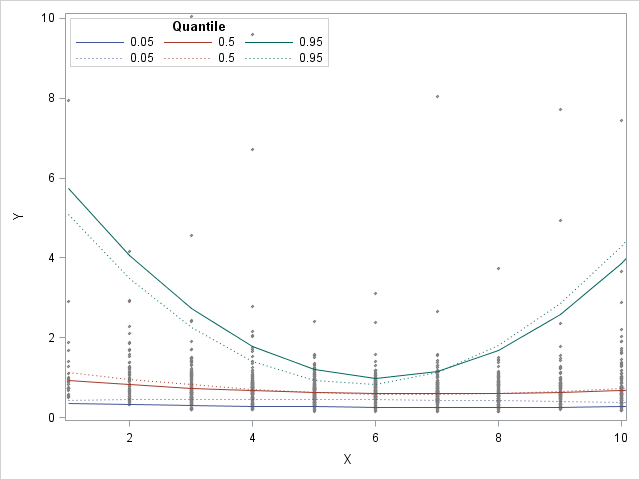## How is it possible to add legendlabels to grouped variables using prog sgplot?

I want to plot the results of two quantile regressions. The output of my quantile regression was a grouped variable "quantile" plus the corresponding values. I tried to create a graph with the following code in SAS 9.4:

``````ods graphics on / labelmax=42100 antialiasmax=42100;
proc sgplot data=data1;
scatter x=Var1 y=Var2;
series x=Var1 y=Var3 / group=quantile legendlabel="Gew" name="G";
series x=Var1 y=Var4 / group=quantile lineattrs=(pattern=dot) legendlabel="OhneGew" name="OG";
keylegend "G" "OG" / location=inside position=topleft title="Quantile" titleattrs=(weight=bold) across=3;
run;
ods graphics off;
``````

The resulting plot is attached. My aim was to label the percentiles in the legend. The first row in the legend should begin with "Gew" and should belong to the first three percentiles (solid line). The second one should start with "OhneGew" and should belong to the other three percentiles (dotted line). Therefore I used the keylegend statement along with the legendlabel statement. But as a result, I only get a legend without the required legendlabels "Gew" and "OhneGew".

How can I modify the legend in order to get the legendlabels?